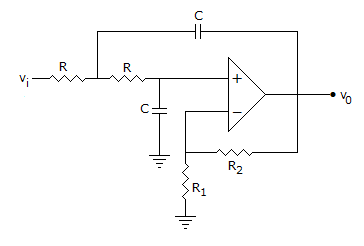# Electronics and Communication Engineering - Analog Electronics

41.

An exponential amplifier has diode in feedback path.

 A. True B. False

Explanation:

It has resistance in feedback path.

42.

DC amplifiers have a tendency to be unstable.

 A. True B. False

Explanation:

Due to drift.

43.

A half wave diode rectifier has a capacitance input filter. If input voltage is Vm sin ωt, PIV is

 A. Vm B. 2 Vm C. 3 Vm D. 4 Vm

Explanation:

Because capacitor charge is retained.

44.

An amplifier with loop gain Aβ will be more stable for value of Aβ as

 A. 0.95 B. 0.7 C. 1.2 D. 1.5

Explanation:

A feedback amplifier is stable if loop gain is less than unity. More less, more stable, hence β will be more stable.

45.

Study the circuit of figure and examine the following statements1. It is an active low pass finer.
2. It is a second order filter.
3. The change in gain is 40 dB/decade.
Which of the above statements are correct?

 A. 1, 2 and 3 B. 1 and 2 C. 1 and 3 D. 2 and 3